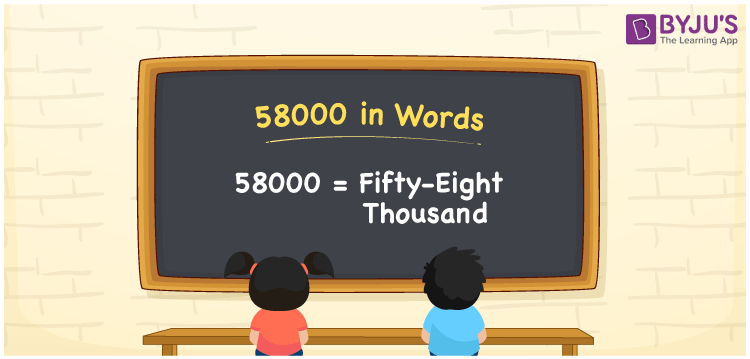# 58000 in Words

58000 in words can be written as Fifty-eight thousand. It is possible to convert 58000 into words using a place value chart easily. If you have contributed Rs. 58000 for school development, you could say, “I contributed Fifty-eight thousand rupees to a school”. Also, 58000 is a cardinal number since it denotes a specific quantity.

 58000 in words Fifty-eight thousand Fifty-eight thousand in Numbers 58000

## 58000 in English Words

We generally write numbers in words with the help of the English alphabet. Therefore, we can read 58000 in English as “Fifty-eight thousand”.## How to Write 58000 in Words?

To write the number 58000 in words, we should make a five-column place chart since it has five digits. Let’s understand the conversion of 58000 to word form from the table below.

 Ten Thousands Thousands Hundreds Tens Ones 5 8 0 0 0

Thus, we can write the expanded form as:

5 × Ten Thousand + 8 × Thousand + 0 × Hundred + 0 × Ten + 0 × One

= Fifty thousand + Eight thousand

= Fifty-eight thousand

Hence, 58000 in words is written as Fifty-eight thousand.

58000 is a natural number that precedes 58001 and succeeds 57999.

58000 in words – Fifty-eight thousand

Is 58000 an odd number? – No

Is 58000 an even number? – Yes

Is 58000 a perfect square number? – No

Is 58000 a perfect cube number? – No

Is 58000 a prime number? – No

Is 58000 a composite number? – Yes

## Frequently Asked Questions on 58000 in Words

Q1

### How do you write 58000 in English words?

We can write the number 58000 in English words as “Fifty-eight thousand”.
Q2

### How do you write 58000 in words on a cheque?

On a cheque, we write 58000 in words as Fifty-eight thousand rupees only.
Q3

### Write the value of 32000 + 26000 in words.

32000 + 26000 = 58000 Hence, the value of 32000 + 26000 is 58000, which can be written in words as Fifty-eight thousand.